Correlations
Predictions
Scatterplots
Line of Best Fit
Correlations
100

Hours of exercise and weight loss

What is positive

100

What was the temperature when the beach had the most visitors?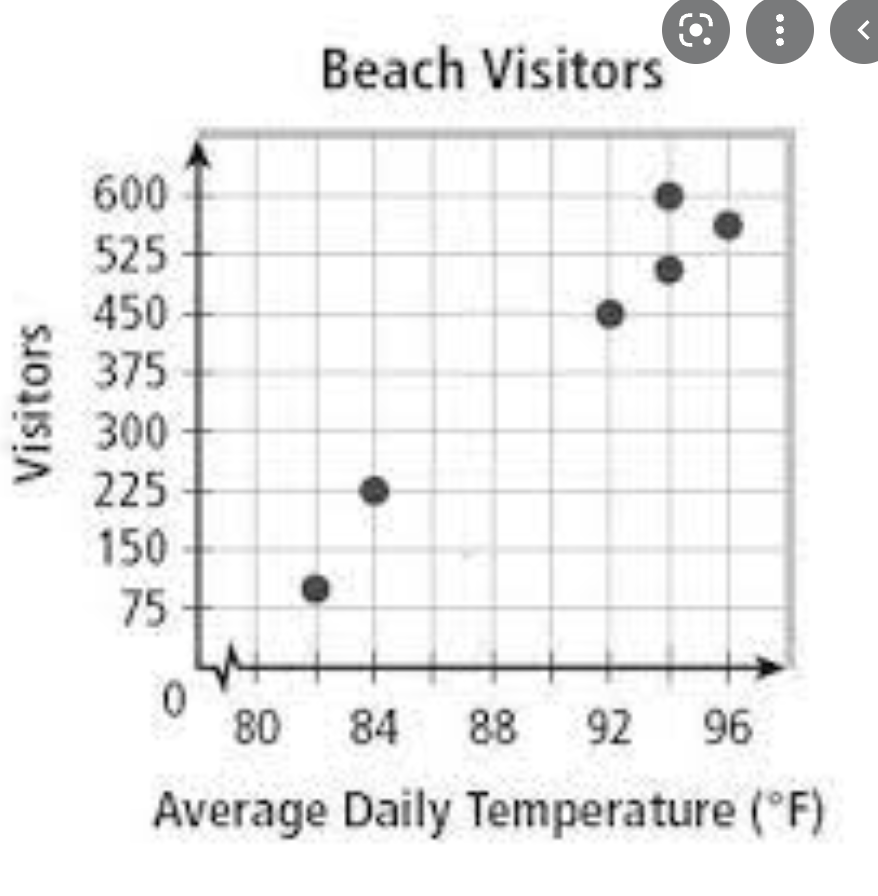94 degrees it had 600 vistors

100

Draw a scatterplot showing a no correlation

Something with dots all over

100

Define or describe a line of best fit.

A line on a graph showing the general direction that a group of points seem to follow.

100

What type of correlation?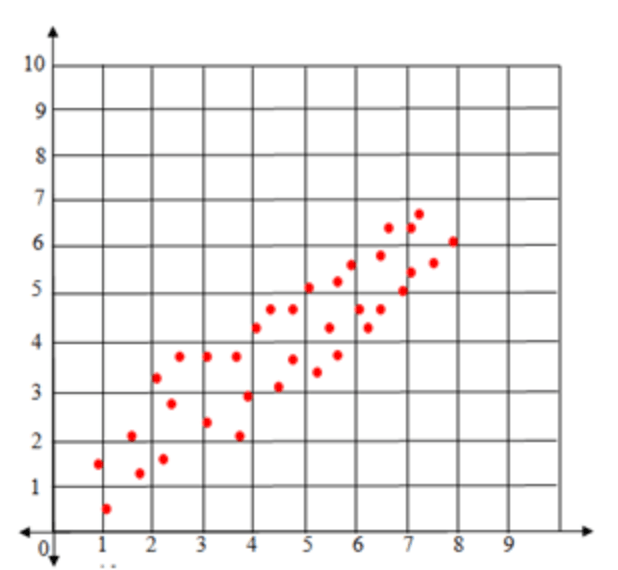Positive Correlation

200

Temperature outside and number of pets

What is no correlation

200

For someone to who studied 1 hour what was their score on the final exam?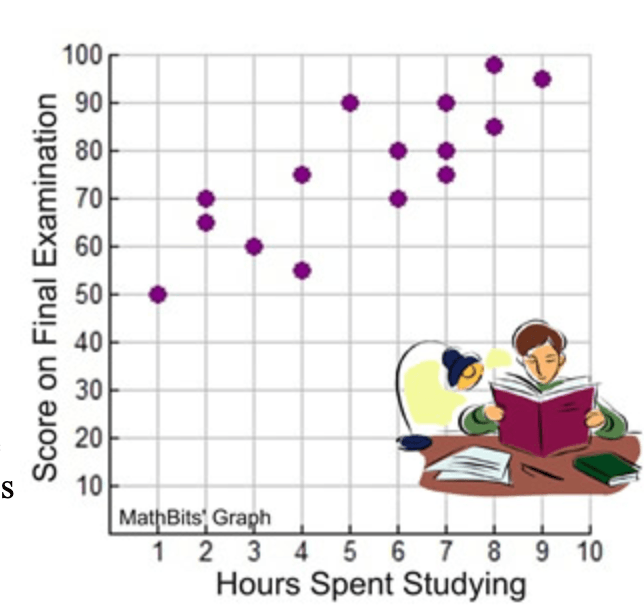50%

200

Draw a scatterplot showing a weak positive correlation

Dots going up from left to right but not close together

200B

200

What type of correlationNegative Correlation

300

Time spent doing homework and number of missing assignments

What is negative

300

What is the score for someone who studied 5 hours?90%

300

Draw a scatterplot showing a strong positive correlation

Dots going up closer together from left to right
300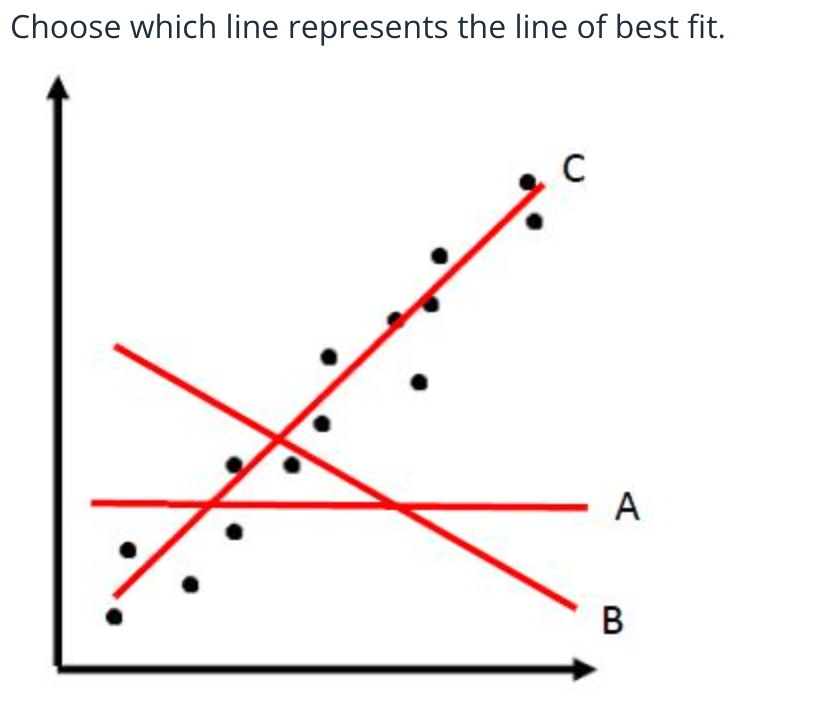C

300

What type of correlation be specific?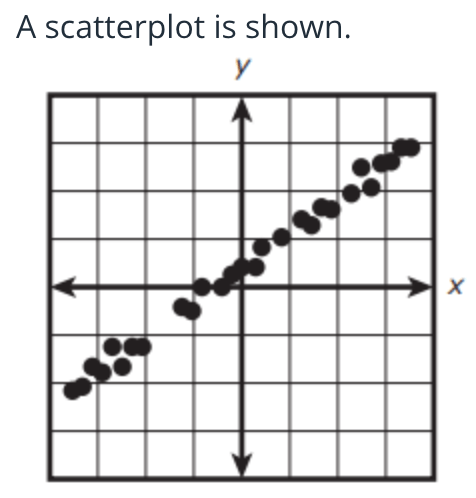Strong Positive Correlation

400

What type of correlation is show in a scatterplot where y tends to increase as x tends to increase?

Positive Correlation

400

If the temperature is 90 degrees about how many visitors should the beach expect?Between 350-400

375 would be the best answer

400

Draw a scatterplot showing a strong negative correlation

Dots going down from left to right close together.

400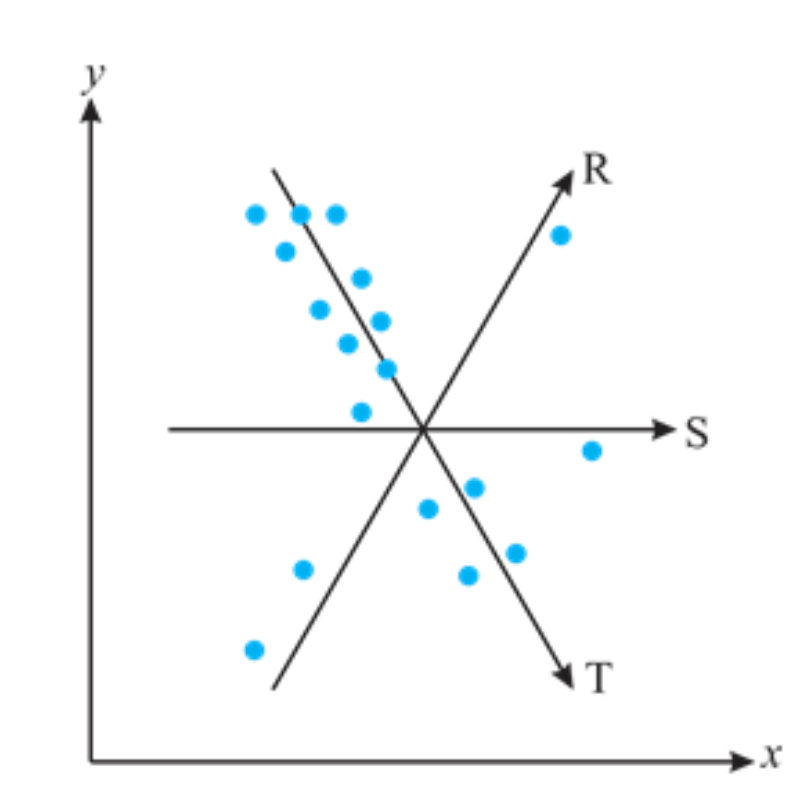Line T

400

Describe the scatterplot below. What is happening in this situation.As the average daily temperature increase, the number of Beach goers also increases.

(As X increases, Y increases)

500

What type of correlation does a scatterplot show where y tends to decrease as x tends to increase?

Negative Correlation

500

How many hours should someone study to get an A or above a 90% on the exam?At least 5 hours

500

Draw a scatterplot showing a weak negative correlation

Dots going down from left to right not close together.

500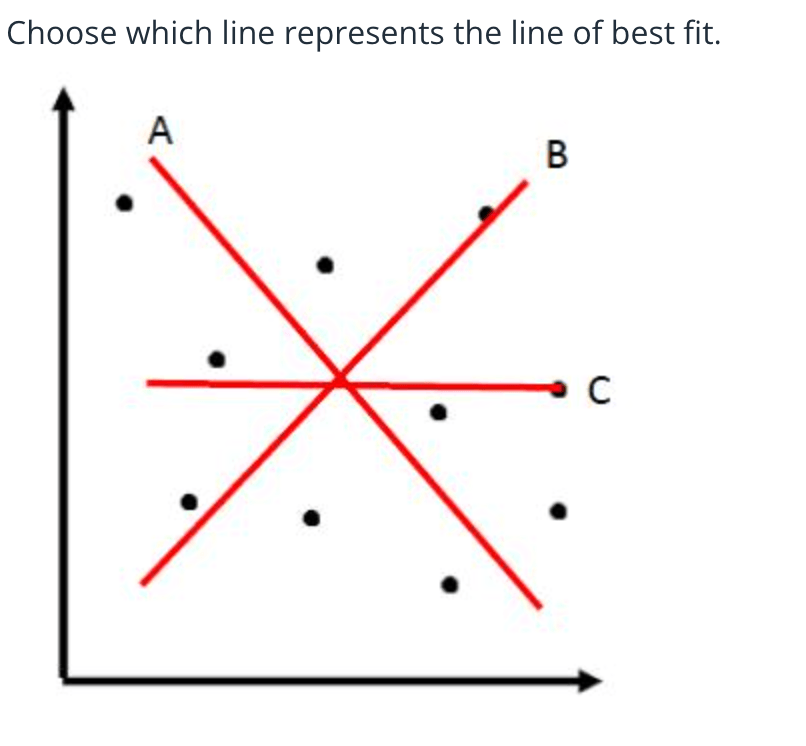There is no correlation there is no line of best fit

500

Describe the scatterplot in relation the the situation.If the student increases the number of study hours their final exam score also increase.

(As X increase, Y increases)

Click to zoom